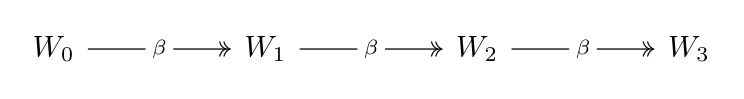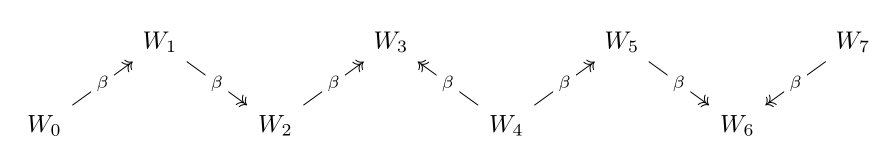Show Question
Math and science::Theory of Computation::Lambda calculus

# β-reduction

Beta reduction is a term that encompasses 3 relations defined over the set of all lambda terms: single-step, multi-step and equality.

### β-reduction, single step, $$\rightarrow_{\beta}$$

Let $$M, U$$ and $$L$$ be lambda terms.

The following 2 rules define the true entries of the single step beta reduction relation.

Basis
$$(\lambda .\, M) U \rightarrow_{\beta} M[x :=U]$$
Compatibility
If $$M \rightarrow_{\beta} U$$, then:
• $$ML \betaReduction UL$$
• $$LM \betaReduction LU$$
• $$\lambda x.\,M \rightarrow_{\beta} \lambda x. U$$

the syntax $$[x :=U]$$ refers to the lambda terms that results from substituting all occurrences of the unbound variable $$x$$ by the term $$U$$. This syntax in some sense requires some "work".

There are names given to parts of the above definition:

#### Redex and contractum

If a subterm of a lambda expression matches the left-hand side of the basis rule above, it is called a redex. This name comes from from reducible expression. The subterm on the right-hand side, $$M[x:=U]$$, is called the contractum of the redex.

Multi-step reduction extends the single step relation by augmenting it with reflexivity and transitivity. Before we get to multi-step reduction, we describe the following definition:

#### Reduction sequence (personal definition)

A reduction sequence is a sequence of lambda terms $$(W_n)_{n=0}^{N}$$ such that for every $$n \in \mathbb{N}, \, n \lt N$$, we have $$W_n \betaReduction W_{n+1}$$. The sequences starts at $$W_0$$ and ends at $$W_N$$.

### β-reduction, multi-step, $$\twoheadrightarrow_{\beta}$$

Let $$M$$ and $$U$$ be lambda terms.

$$M$$ reduces through multi-step β-reduction to $$U$$ iff there exists a reduction sequence starting at $$M$$ and ending at $$U$$.

Note: the possibility of $$n = 0$$ for multi-step reduction is what introduces the quality of reflexivity to the relation.

For multi-step β-reduction, it is possible for $$M \rightarrow_{\beta} U$$ to be true while $$U \rightarrow_{\beta} M$$ is false. Below, we extend the multi-step β-reduction with symmetry and arrive at β-equality, an equivalence relation. First, another assisting definition.

#### Bi-directional reduction sequence (personal definition)

A bi-directional reduction sequence is a sequence of lambda terms $$(W_n)_{n=0}^{N}$$ such that for every $$n \in \mathbb{N}, \, n \lt N$$ either $$W_n \betaReduction W_{n+1}$$ or $$W_{n+1} \betaReduction W_{n}$$.

### β-equality, $$=_{\beta}$$

Let $$M$$ and $$U$$ be lambda terms.

$$M$$ and $$U$$ are said to be β-equal iff there exists a bi-directional reduction sequence starting at $$M$$ and ending at $$U$$.

β-convertable is sometimes said instead of β-equal.

### β-equality extends β-reduction in both directions

If $$M \twoheadrightarrow_{\beta} U$$ or $$U \twoheadrightarrow_{\beta} M$$ then $$M =_{\beta} U$$.

The reverse statement is not true.

### Graphs of reduction sequences

The following graph shows a possible reduction sequence that implies that $$W_0 \multiBetaReduction W_3$$ is true. $$W_0$$ multi-step reduces to $$W_0$$, $$W_1$$ and $$W_2$$ also.The next graph shows a bi-directional reduction sequence that implies that $$W_0 \betaEq W_5$$ is true. In fact, all nodes are β-equal to each other.The nodes are in a zig-zag pattern simply to condense the diagram and this layout is not intended to convey any other meaning.

## Context

β-reduction → β-normal form → confluence